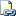# SOME | ANY (Transact-SQL)

Compares a scalar value with a single-column set of values. SOME and ANY are equivalent.Transact-SQL Syntax Conventions

## Syntax

``````scalar_expression { = | < > | ! = | > | > = | ! > | < | < = | ! < }
{ SOME | ANY } ( subquery )
``````

## Arguments

• scalar_expression
Is any valid expression.

• { = | <> | != | > | >= | !> | < | <= | !< }
Is any valid comparison operator.

• SOME | ANY
Specifies that a comparison should be made.

• subquery
Is a subquery that has a result set of one column. The data type of the column returned must be the same data type as scalar_expression.

Boolean

## Result Value

SOME or ANY returns TRUE when the comparison specified is TRUE for any pair (scalar_expression**,** x) where x is a value in the single-column set; otherwise, returns FALSE.

## Remarks

SOME requires the scalar_expression to compare positively to at least one value returned by the subquery. For statements that require the scalar_expression to compare positively to every value that is returned by the subquery, see ALL (Transact-SQL). For instance, if the subquery returns values of 2 and 3, scalar_expression = SOME (subquery) would evaluate as TRUE for a scalar_express of 2. If the subquery returns values of 2 and 3, scalar_expression = ALL (subquery) would evaluate as FALSE, because some of the values of the subquery (the value of 3) would not meet the criteria of the expression.

## Examples

### A. Running a simple example

The following statements create a simple table and add the values of 1, 2, 3, and 4 to the ID column.

``````CREATE TABLE T1
(ID int) ;
GO
INSERT T1 VALUES (1) ;
INSERT T1 VALUES (2) ;
INSERT T1 VALUES (3) ;
INSERT T1 VALUES (4) ;
``````

The following query returns TRUE because 3 is less than some of the values in the table.

``````IF 3 < SOME (SELECT ID FROM T1)
PRINT 'TRUE'
ELSE
PRINT 'FALSE' ;
``````

The following query returns FALSE because 3 is not less than all of the values in the table.

``````IF 3 < ALL (SELECT ID FROM T1)
PRINT 'TRUE'
ELSE
PRINT 'FALSE' ;
``````

### B. Running a practical example

The following example creates a stored procedure that determines whether all the components of a specified SalesOrderID in the AdventureWorks2012 database can be manufactured in the specified number of days. The example uses a subquery to create a list of the number of DaysToManufacture value for all the components of the specific SalesOrderID, and then tests whether any of the values that are returned by the subquery are greater than the number of days specified. If every value of DaysToManufacture that is returned is less than the number provided, the condition is TRUE and the first message is printed.

``````USE AdventureWorks2012 ;
GO

CREATE PROCEDURE ManyDaysToComplete @OrderID int, @NumberOfDays int
AS
IF
@NumberOfDays < SOME
(
SELECT DaysToManufacture
FROM Sales.SalesOrderDetail
JOIN Production.Product
ON Sales.SalesOrderDetail.ProductID = Production.Product.ProductID
WHERE SalesOrderID = @OrderID
)
PRINT 'At least one item for this order cannot be manufactured in specified number of days.'
ELSE
PRINT 'All items for this order can be manufactured in the specified number of days or less.' ;
``````

To test the procedure, execute the procedure by using the SalesOrderID 49080, which has one component that requires 2 days and two components that require 0 days. The first statement meets the criteria. The second query does not.

``````EXECUTE ManyDaysToComplete 49080, 2 ;
``````

Here is the result set.

All items for this order can be manufactured in the specified number of days or less.

``````EXECUTE ManyDaysToComplete 49080, 1 ;
``````

Here is the result set.

At least one item for this order cannot be manufactured in specified number of days.

#### Reference

ALL (Transact-SQL)

CASE (Transact-SQL)

Built-in Functions (Transact-SQL)

Operators (Transact-SQL)

SELECT (Transact-SQL)

WHERE (Transact-SQL)

IN (Transact-SQL)